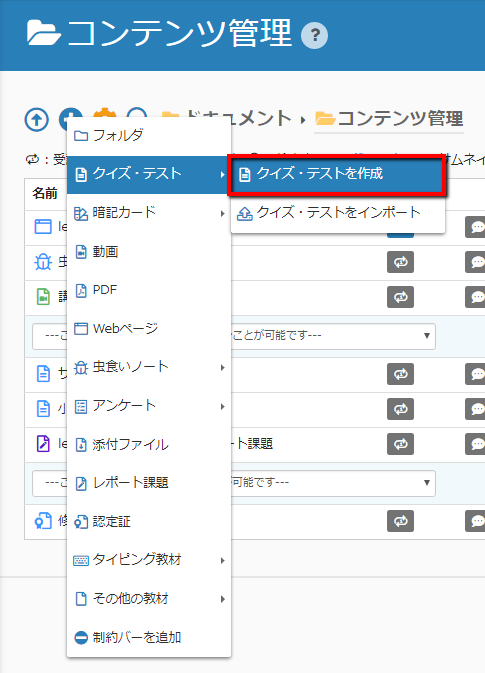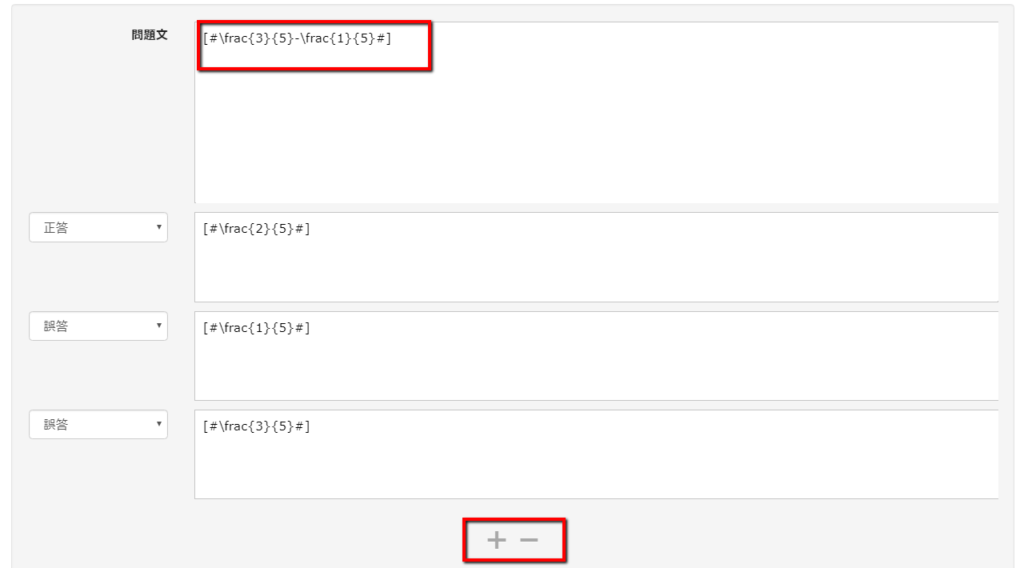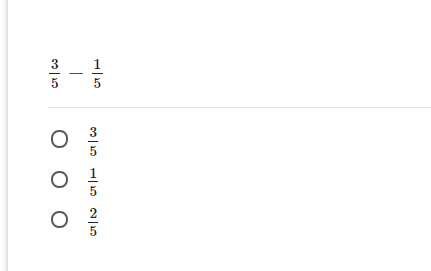# Insert a Math Formulas

• HOME
• Insert a Math Formula

### Insert Math Formula

QuizGenerator can create questions using mathematical formulas. If you write a mathematical expression in LaTeX between [# and #], it will be converted into an image and displayed in the question. You can use it within the commentary. Please refer to the “LaTeX command sheet” list etc for how to use LaTeX

In addition, you can check the examples of the input of the formula in LaTeX format from the following site.

Check the Latex format

### How to create the mathematical formulas

• Text
• Excel
• Quiz Creation Form

#passing_score:50
#time_limit:300
#messages_intro:Start the quiz!
What is a total sum of interior angles of pentagon?
sa:
[#540^\circ#] [#360^\circ#] [#450^\circ#] [#(3\times3)-(6\div2)#] sa:
6
3
2

[#3^{3}-2^{3}#] sa:
19
13
5

[#(6\times7)-(25\div5)#] sa:
37
27
17

What is a total sum of interior angles of parallelogram?
sa:
[#105^\circ#] [#205^\circ#] [#115^\circ#] [#(8\times8)-(72\div9)#] sa:
56
46
66

[#\frac{3}{5}-\frac{1}{5}#] sa:
[#\frac{2}{5}#] [#\frac{1}{5}#] [#\frac{3}{5}#]

 A B C D E 1 #format:table sa: 2 What is a total sum of interior angles of pentagon? sa: [#540^\circ#] [#360^\circ#] [#450^\circ#] 3 [#(3\times3)-(6\div2)#] sa: 6 3 2 4 [#3^{3}-2^{3}#] sa: 19 13 5 5 [#-8+\{3-(-2^{3})\}\times4-(4^{2}+5)\div(-3)#] sa: 43 32 37 6 What is the root of the next numbers. sa: [#\pm484#] [#\pm22#] [#\pm18#]

### Sample

You can create the question using the math formula

If you write a formula in the LaTeX format between [# and #] like the red frame in the following quiz, it will be converted into the image and displayed in the question. You can use it within the commentary. Please refer to the LaTeX command sheet list etc. for how to use LaTeX.

Click the “Content Management” menu button (+ button) → “Test/Quiz” → “Create a Test/Quiz”.Please click on “Edit” and select the question format.

Enter the question sentences and options in LaTeX format.
You can add an option with the “+” button in the red frame or delete an option with the “-” button.Completed!